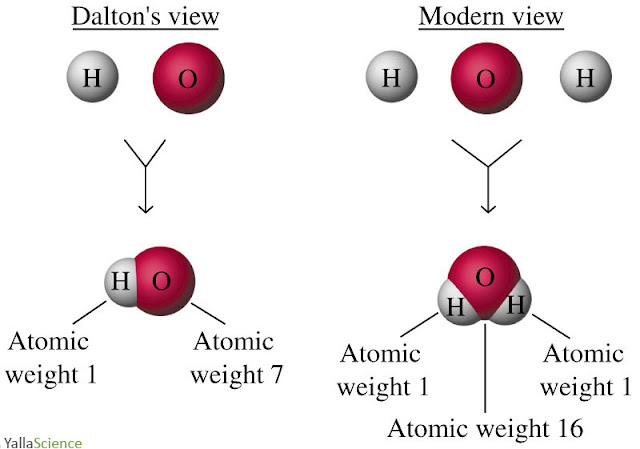# Chemistry Dictionary

## DonateName

Email *

Message *

## Dalton's atomic weight problems

Dalton assumed a combining ratio of hydrogen to oxygen atoms of 1:1.
Data at the time suggested that the mass ratio of hydrogen to oxygen in water was 1:7. Taking the atomic weight of hydrogen to be one, that of oxygen was seven.

Modern data indicate that the combining ratio of hydrogen to oxygen atoms is 2:1 and that the mass ratio is 1:8 (or 2:16).

If the atomic weight of hydrogen is taken to be one, that of oxygen must be 16.

see also: Dalton's theory and conservation of massDalton's atomic weight problems

Tage:

 the law of multiple proportions, Dalton, atomic mass, water, mass percent
Source:
Freefullpdf for Scientific Publications